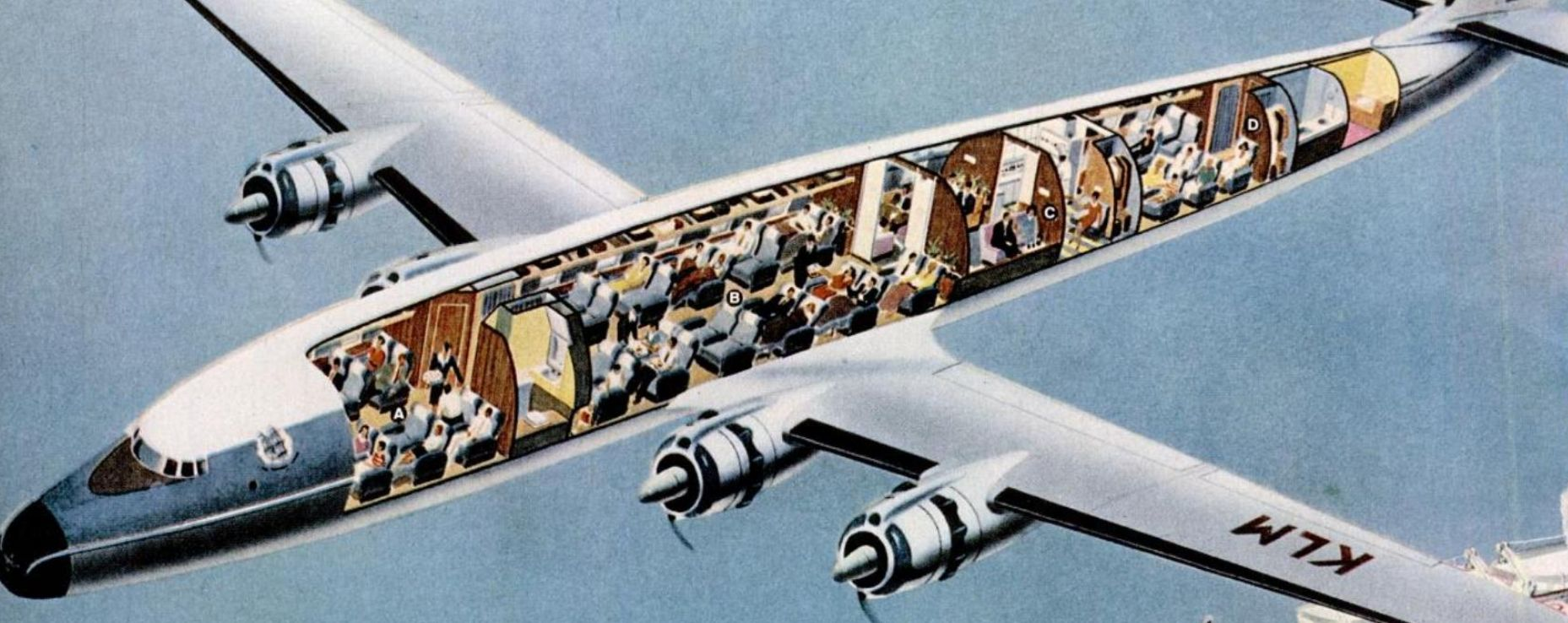# Have you ever thought your airline ticket cost too much?Suppose you're flying from San Francisco (SFO) to New York (NYC). The plane (Airbus 320) carries 189 passengers and has 6 crew members who each make $20 per hour. Assume a minimal model where the only costs of operating the flight are paying the salary of the crew, and paying for fuel. Further, assume that the all the engine does is fight the drag force. If each ticket costs$300, jet fuel costs \$0.75 per liter, and the crew is paid for the number of complete hours they work, what percent of your ticket price goes toward the airline's profit?

Assumptions and Details

• The Airbus has a drag coefficient $C_D$ of $\frac{1}{3\pi}.$
• The density of air is $\rho=1.275\text{ kg/m}^3$.
• The plane flies at $511\text{ mph } (228\text{ m/s})$ from start to finish.
• The distance from NYC to SFO is $4148\text{ km}.$
• The energy efficiency of the jet engines is $\eta=16\%$.
• Approximate the Airbus as a cylinder of diameter $6\text{ m}.$
• The energy density of the jet fuel is $45\times 10^6\text{ J/kg}.$
• The density of the fuel is $804\text{ kg/m}^3$.
• The crew is paid for each full hour they work, i.e. if they work $5.2\text{ hrs}$ they are paid for $5.$
×